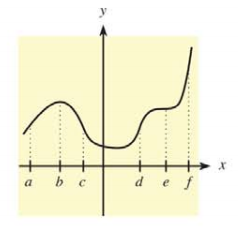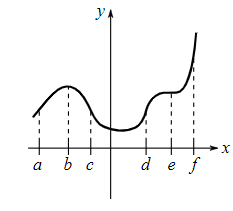Chapter 10.2, Problem 4E### Mathematical Applications for the ...

11th Edition
Ronald J. Harshbarger + 1 other
ISBN: 9781305108042

#### Solutions

Chapter
Section### Mathematical Applications for the ...

11th Edition
Ronald J. Harshbarger + 1 other
ISBN: 9781305108042
Textbook Problem

# In Problems 5-10, use the indicated x-values on the graph of y = f ( x ) to find the following.Find intervals over which the graph is concave up.

To determine

The intervals over which the provided graph is concave up.Explanation

Given Information:

The provided graph is,

Explanation:

Consider the provided graph,

The slope of function f(x) is increasing in the interval (c,d) and second derivative f(x) of function f(x) is positive in the interval (c,d). Therefore, the graph of function f(x) is concave up on an interval (c,d)

### Still sussing out bartleby?

Check out a sample textbook solution.

See a sample solution

#### The Solution to Your Study Problems

Bartleby provides explanations to thousands of textbook problems written by our experts, many with advanced degrees!

Get Started

#### What steps do you follow to graph a polynomial function P?

Precalculus: Mathematics for Calculus (Standalone Book)

#### let f(x) = x3 + 5, g(x) = x2 2, and h(x) = 2x + 4. Find the rule for each function. 8. fgh

Applied Calculus for the Managerial, Life, and Social Sciences: A Brief Approach

#### Evaluate the expression sin Exercises 116. (23)2

Finite Mathematics and Applied Calculus (MindTap Course List)

#### True or False: n=1(1)nn+14 is a convergent series.

Study Guide for Stewart's Single Variable Calculus: Early Transcendentals, 8th

#### What can a researcher do if the treatment administered during the first B?

Research Methods for the Behavioral Sciences (MindTap Course List)